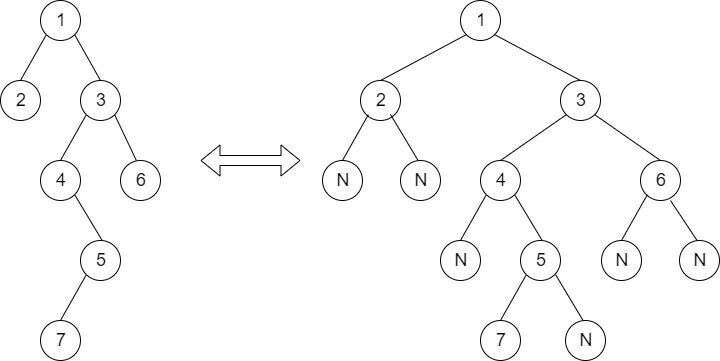Timer is Running

Size of Binary Tree
##### Submissions: 26485   Accuracy: 60.47%   Difficulty: Basic   Marks: 1

Given a binary tree of size N, you have to count number of nodes in it. For example, count of nodes in below tree is 4.

1
/      \
10      39
/
5

Input:
First line of input contains the number of test cases T. For each test case, there will be only a single line of input which is a string representing the tree as described below:

1. The values in the string are in the order of level order traversal of the tree where, numbers denote node values, and a character “N” denotes NULL child.

2. For example:For the above tree, the string will be: 1 2 3 N N 4 6 N 5 N N 7 N

Output:
For each testcase in new line, print the number of nodes.

Since this is a functional problem you don't have to worry about input, you just have to complete the function getSize().

Constraints:
1 <= T <= 30
1 <= N <= 104
Example:
Input:

2
1 2 3
10 5 9 N 1 3 6

Output:
3
6

Explanation:
Testcase 2:
Given Tree is :

10
/        \
5          9
\       /     \
1    3       6
There are six nodes in the tree .

#### ** For More Input/Output Examples Use 'Expected Output' option **

Author: kartik

If you have purchased any course from GeeksforGeeks then please ask your doubt on course discussion forum. You will get quick replies from GFG Moderators there.